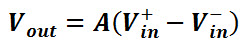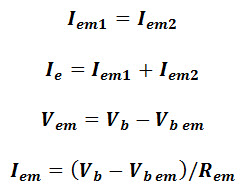# Differential Amplifier Circuit using Transistors

The operational amplifiers are shortly termed as Op-Amps and are also called as differential amplifiers. The operational amplifier is typically used as a differential amplifier in various electrical and electronic circuits. These operational amplifiers can be used for performing filtering, signal conditioning, and mathematical operations. The electrical and electronic components such as resistors and capacitors are used at the input or/and output terminals of the operational amplifier. Such that, the amplifier function results, the benefit of the resistive feedback, or capacitive feedback configurations are regulated by these components. Thus, the amplifier can achieve various operations, hence, it is termed as an operational amplifier. This article discusses an overview of the differential amplifier circuit and its working

## What is Differential Amplifier

The electronic amplifier used for amplifying the difference between two input signals can be called as a differential amplifier. In general, these differential amplifiers consist of two terminals namely inverting terminal and non-inverting terminal. These inverting and non-inverting terminals are represented with – and + respectively.

### Differential Amplifier Circuit

The differential amplifier can be considered as an analog circuit that consists of two inputs and one output. The differential amplifier circuit can be represented as shown in the figure below.

The output voltage of a differential amplifier is proportional to the difference between the two input voltages. This can be represented in equation form as follows:Where A= gain of the amplifier.

### Differential Amplifier Circuit using Transistors

The differential amplifier circuit using transistors can be designed as shown in the figure below which consists of two transistors T1 and T2. These transistors and resistors are connected as shown in the circuit diagram.

There are two inputs I1 & I2 and two outputs V1out & V2out in the differential amplifier circuit. The input I1 is applied to the transistor T1 base terminal, input I2 is applied to the transistor T2 base terminal. The emitter terminals of transistor T1 and transistor T2 are connected to a common emitter resistor. Thus, the two input signals I1 & I2 will affect the outputs V1out & V2out. The differential amplifier circuit consists of two supply voltages Vcc and Vee but there is no ground terminal. Even with single voltage supply also circuit can be operated fine as it is intended (similarly while using two supply voltages). Hence, the opposite points of positive voltage supply & negative voltage supply are connected to the ground.

### Working

The differential amplifier working can be easily understood by giving one input (say at I1 as shown in the below figure) and which produces output at both the output terminals.

If the input signal (I1) is supplied to the base of transistor T1, then a high voltage drop appears across the resistor connected to the transistor T1 collector terminal which will get less positive. If no input signal (I1) is supplied to the base of transistor T1, then a low voltage drop appears across the resistor connected to the transistor T1 collector terminal which will get more positive. Thus, we can say that the inverting output appearing across the collector terminal of transistor T1 is based on the input signal I1 supplied at the base terminal of T1.

If T1 is turned ON by applying the positive value of I1, then the current passing through emitter resistance increases as the emitter current & collector current is almost equal. Thus, if the voltage drops across emitter resistance increases, then the emitter of both the transistors goes in a positive direction. If the transistor T2 emitter is positive, then the base of T2 will be negative and in this condition, current conduction is less.

Thus, there will be less voltage drop across the resistor connected at the collector terminal of transistor T2. Hence, for the given positive input signal collector of T2 will go in a positive direction. Thus, we can say that the non-inverting output appearing across the collector terminal of transistor T2 is based on the input signal applied at the base of T1.

The amplification can be driven differentially by taking output between the collector terminals of transistors T1 and T2. From the above circuit diagram, assuming all the characteristics of transistors T1 & T2 are identical and if base voltages Vb1 is equal to Vb2 (base voltage of transistor T1 is equal to base voltage of transistor T2), then emitter currents of both transistors will be equal (Iem1=Iem2). Thus, the total emitter current will be equal to the sum of emitter currents of T1 (Iem1) and T2 (Iem2).

Thus, emitter current can be driven asThus, the emitter current remains constant independent of the hfe value of transistors T1 and T2. If resistances connected to the collector terminals of T1 & T2 are equal, then their collector voltages are also equal.

### Applications

The applications of differential amplifiers include the following.

There are numerous differential amplifier applications in practical circuits, signal amplification applications, controlling of motors & servo motors, input stage emitter-coupled logic, switch, and so on are common applications of the differential amplifier circuit.

For more information regarding amplifier circuits and differential amplifier applications, you can approach us by posting your queries, suggestions, ideas, comments, and also know how to design electronics projects on your own in the comments section below.